• +91 9971497814
• info@interviewmaterial.com

# RD Chapter 21- Some Special Series Ex-21.1 Interview Questions Answers

### Related Subjects

Question 1 : Find the sum of the following series to n terms:
13 + 33 + 53 +73 + ……..

Let Tn bethe nth term of the given series.

We have:

Tn =[1 + (n – 1)2]3

= (2n – 1)3

= (2n)3 –3 (2n)2. 1 + 3.12.2n-1[Since, (a-b)3 =a3 – 3a2b + 3ab2 – b]

= 8n3 –12n2 + 6n – 1

Now, let Sn bethe sum of n terms of the given series.

We have: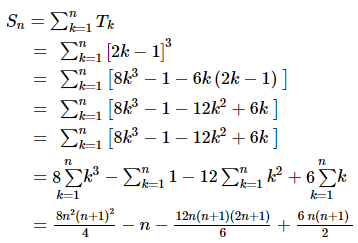Upon simplification weget,

= 2n2 (n+ 1)2 – n – 2n (n + 1) (2n + 1) + 3n (n + 1)

= n (n + 1) [2n (n +1) – 2 (2n + 1) + 3] – n

= n (n + 1) [2n2 –2n + 1] – n

= n [2n3 –2n2 + n + 2n2 – 2n + 1 – 1]

= n [2n3 –n]

= n2 [2n2 –1]

The sum of the seriesis n2 [2n2 – 1]

Question 2 :

23 + 43 + 63 + 83 +………

Let Tn bethe nth term of the given series.

We have:

Tn =(2n)3

= 8n3

Now, let Sn bethe sum of n terms of the given series.

We have: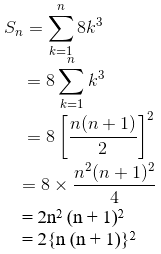The sum of the seriesis 2{n (n + 1)}2

Question 3 :

1.2.5 + 2.3.6 + 3.4.7 + ……..

Let Tn bethe nth term of the given series.

We have:

Tn = n(n + 1) (n + 4)

= n (n2 +5n + 4)

= n3 +5n2 + 4n

Now, let Sn bethe sum of n terms of the given series.

We have: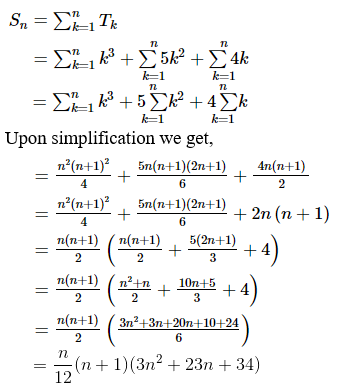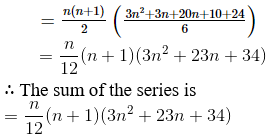Question 4 :

1.2.4 + 2.3.7 + 3.4.10 + … to n terms.

Let Tn bethe nth term of the given series.

We have:

Tn = n(n + 1) (3n + 1)

= n (3n2 +4n + 1)

= 3n3 +4n2 + n

Now, let Sn bethe sum of n terms of the given series.

We have: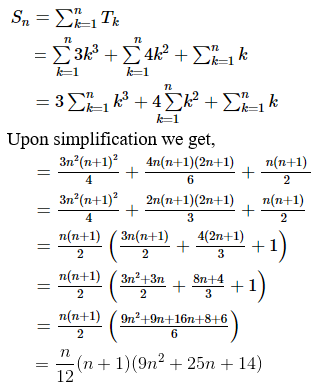The sum of the seriesis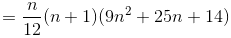Question 5 :

1 + (1 + 2) + (1 + 2 + 3) + (1 + 2 + 3 + 4) + … to n terms

Let Tn bethe nth term of the given series.

We have:

Tn =n(n+1)/2

= (n2 +n)/2

Now, let Sn bethe sum of n terms of the given series.

We have: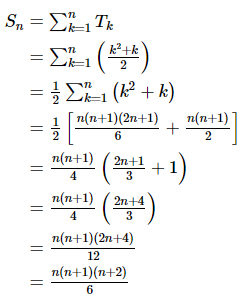The sum of the seriesis [n(n+1)(n+2)]/6

Todays Deals### RD Chapter 21- Some Special Series Ex-21.1 Contributorskrishan

Name:
Email:

# Latest News# 9000 interview questions in different categories